×
Get Full Access to Statistics For Business And Economics - 12 Edition - Chapter 3 - Problem 32e
Get Full Access to Statistics For Business And Economics - 12 Edition - Chapter 3 - Problem 32e

×

# Consider the Venn diagram in the next column, whereP(E1) =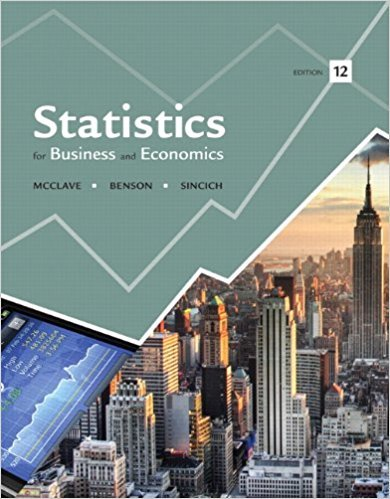ISBN: 9780321826237 51

## Solution for problem 32E Chapter 3

Statistics for Business and Economics | 12th Edition

• Textbook Solutions
• 2901 Step-by-step solutions solved by professors and subject experts
• Get 24/7 help from StudySoup virtual teaching assistantsStatistics for Business and Economics | 12th Edition

4 5 1 294 Reviews
15
4
Problem 32E

Problem 32E

Consider the Venn diagram in the next column, where

P(E1) = .10, P(E2) = .05, P(E3) = P(E4) = .2, P(E5) = .06, P(E6) = .3, P(E7) = .06, and P(E8) = .03.

Find the following probabilities:

a. P(Ac)

b. P(Bc)

c. P(Ac B)

d. P(A B)

e. P(AB)

f. P(AcBc)

g. Are events A and B mutually exclusive? Why?Step-by-Step Solution:

Step by Step Solution

Step 1 of 8

We have: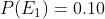,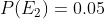,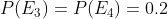,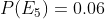,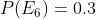,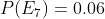and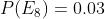.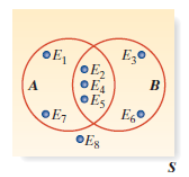Step 2 of 8

Step 3 of 8

##### ISBN: 9780321826237

Statistics for Business and Economics was written by and is associated to the ISBN: 9780321826237. This textbook survival guide was created for the textbook: Statistics for Business and Economics , edition: 12. Since the solution to 32E from 3 chapter was answered, more than 1457 students have viewed the full step-by-step answer. The answer to “Consider the Venn diagram in the next column, whereP(E1) = .10, P(E2) = .05, P(E3) = P(E4) = .2, P(E5) = .06, P(E6) = .3, P(E7) = .06, and P(E8) = .03.Find the following probabilities:a. P(Ac)b. P(Bc)c. P(Ac B)d. P(A ? B)e. P(A B)f. P(Ac Bc)g. Are events A and B mutually exclusive? Why?” is broken down into a number of easy to follow steps, and 54 words. The full step-by-step solution to problem: 32E from chapter: 3 was answered by , our top Business solution expert on 07/21/17, 05:42AM. This full solution covers the following key subjects: column, consider, diagram, Events, exclusive. This expansive textbook survival guide covers 15 chapters, and 1631 solutions.

Unlock Textbook Solution